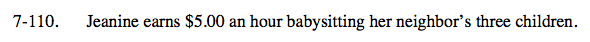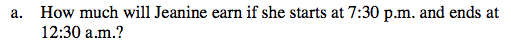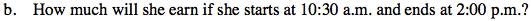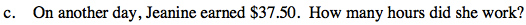### Home > CC1 > Chapter 7 > Lesson 7.3.3 > Problem7-110

7-110.
1. Jeanine earns $5.00 an hour babysitting her neighbor’s three children. Homework Help ✎ 1. How much will Jeanine earn if she starts at 7:30 p.m. and ends at 12:30 a.m.? 2. How much will she earn if she starts at 10:30 a.m. and ends at 2:00 p.m.? 3. One day, Jeanine earned$37.50. How many hours did she work?How many hours did Jeanine work? $5.00(number of hours) = total earningsJeanine worked 3.5 hours.$\frac{37.50}{5}=?$ Can you do this in your head?$2.50 is half of $5.00.$35.00 is $2.50 less than$37.50.